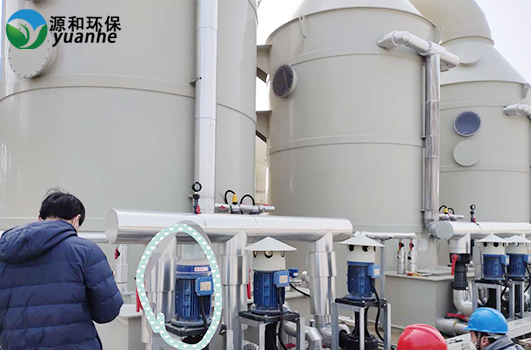﻿ 喷淋塔废气处理-部分废气填料层高度计算

喷淋塔废气处理-部分废气填料层高度计算○一小时流入洗净塔的气体质量

(气体温度60℃時) G'(60℃) = 500m3/分 ×(273K/333K)×1.29㎏/m3(空气密度) ×60分/hr

G'(60℃) = 31,727 kg/hr

(气体温度20℃時)G'(20℃) = 36,058 kg/hr

○洗净塔断面积 (2200 m/2) × (2200 m/2) ×π= 3.799 m2

◎气体质量速度

(1)現状(充填材高度2000mm)、推定的处理后的氰气浓度

2.00 m　　= 0.38 m ×Ln( 10 ppm ÷ X ppm)

Ln( 10 ÷ X = 5.263

Ln(X) = LN(10)- 5.263= 2.303 - 5.263= -2.961

?ogeX = -2.961

X = e3.677= 0.052

(2)为使气体检出值达到界限以下，填充材的高度计算：

0.12mg/Nm3　=0.1ppm

Y m　 =0.38 m ×Ln(10 ppm ÷ 0.1ppm)

=0.38 m ×Ln(100 )=0.38 m ×4.605=1.75 m

1.75 m　× 120%= 2.1 m

○一小时流入洗净塔的气体质量

(气体温度60℃時) G'(60℃) = 500m3/分 ×(273K/333K)×1.29㎏/m3(空気密度) × 60分/hr

G'(60℃) = 31,727 kg/hr

(气体温度20℃時) G'(20℃) = 36,058 kg/hr

○洗净塔断面积 ( 2200 m/2) × ( 2200 m/2) × π= 3.799 m2

◎气体质量速度

1.对于NH3气体的性能

(1)处理气体浓度达标的填充材高度

NoG=Ln( 1500 ppm ÷30 ppm)=3.912

120% × 0.38 m × 3.912≈ 1.8 m

(2)根据现状(填充材高度2000mm)、推定处理后的氨气浓度

2.00 m　　= 0.38 m ×Ln( 1500 ppm ÷ X ppm) ，得出：

Ln(1500 ÷ X)　=5.263

Ln(X)　=LN(1500)-5.263=7.313 -5.263=2.050

?ogeX　=2.050

X　=e3.677=7.768

(3)填充材高度设为1800mm的时候，推测处理后的氨气浓度

1.8 m　　= 0.38 m ×Ln( 1500 ppm ÷ X ppm) ，得出：

Ln(1500 ÷ X)　=4.694

Ln(X)　=LN(1500)-4.694=7.313 -4.694=2.619

X 　= e3.677 = 13.719

“推荐阅读”

【本文标签】：喷淋塔废气处理
【责任编辑】：源和环保版权所有：http://www.yuanhe-ks.com转载请注明出处